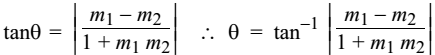# Angle Between Two Straight Lines

Let L1 and L2 be the two intersecting lines and assume that P be the point of intersection of the two straight lines which makes angle θ1 and θ2 with the positive direction of x-axis.L1: y = m1x + c1

L2: y = m2x + c2

m1 = tan θ1

m2 = tan θ2

Let θ be the angle between the two straight lines.

θ1 = θ + θ2

θ = θ1 − θ2

tan θ = tan (θ1 − θ2)### Parallel Lines

If the two straight lines are parallel, then their slopes are equal.

m1 = m2

If the straight lines are parallel, then the coefficients of x and y are proportional in their equations. In particular, the equations of two parallel straight lines differ only by the constant term.

### Perpendicular Lines

If the two straight lines are perpendicular then the product of their slopes is −1.

1 + m1m2 = 0

m1m2 = −1

The equation of the straight line perpendicular to the straight line ax + by + c = 0 is of the form bx − ay + k = 0 for some k.